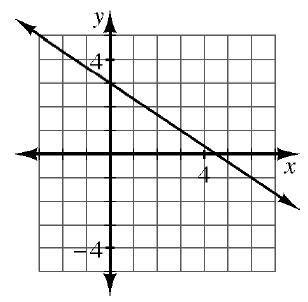### Home > GC > Chapter 2 > Lesson 2.2.1 > Problem2-65

2-65.Examine the graph at right.

1. Find the equation of the line.

What are the slope and $y\text{-intercept}$?

2. Is the line $y=\frac{3}{2}x+1$ perpendicular to this line? How do you know?

Yes, it is perpendicular because the slopes are opposite reciprocals.

3. On graph paper, graph $\overleftrightarrow{AB}$ if $A(-2,4)$ and $B(4,7)$. Then find the equation of $\overleftrightarrow{AB}$.

Graph the points. What are the slope and $y\text{-intercept}$?

$y=0.5x+5$

4. Find an equation of $\overleftrightarrow{AC}$ if $\overleftrightarrow{AC}\perp\overleftrightarrow{AB}$ from part (c).

Many answers are possible depending on your choice of $y\text{-intercept}$. Your slope should be $-2$.